# High-Pass Low-Pass Phase Shifters

Click here to go to our page on switched filter phase shifters

New for March 2016: Thanks to Avinash Sharma, we now have a derivation of the equations for high-pass/low-pass filters used in phase shifters!

On this page we show the exact calculations for tee and pi lumped element filters to achieve any phase shift for any frequency and characteristic impedance.

If a constant phase shift is desired over a wide frequency range, the switched line phase shifter isn't going to cut it. A high-pass/low-pass phase shifter can provide near constant phase shift over an octave or more. By "high-pass/low-pass" we refer to the fact that one arm forms a high-pass filter while the opposite arm forms a low-pass filter. A second advantage of the high-pass/low-pass phase shifter is that it offers a very compact layout because lumped elements are typically used instead of delay lines. This is an important consideration for "low frequency" designs (i.e. below X-band) because delay transmission lines can be quite large. The cutoff frequencies of the two filter networks obviously must be outside of the phase shift band for this scheme to work.

The figure below shows predicted phase response for a high-pass/low-pass 90-degree phase lumped-element shifter optimized for C-band operation.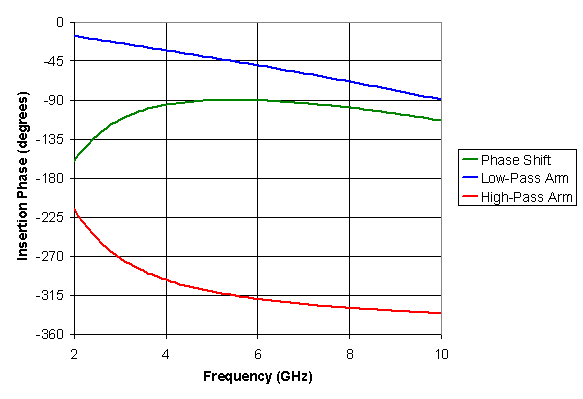High-pass/low-pass phase shifter, C-band 90 degree bit

Referring to the schematic below, the values of the lumped elements in the simulation are C1=1.40 pF, L1=2.09 nH, C2=0.40 pF, and L2=0.63 nH; these were determined using the optimizer within Agilent's ADS simulator to achieve the flattest phase response from 4 to 8 GHz. This is an ideal phase shifter, switch insertion phase contributions were neglected in the simulation. High-pass/low-pass phase shifters usually require two control signals to throw the switches (we'll discuss some that don't need two switches one of these days). Between 4 and 8 GHz the phase shift (green trace) stays within -90 to -99 degrees, a nearly flat response that is not possible from a switched line phase shifter. By our Microwaves101 convention, the high-pass arm provides the reference state, as it provides the least-negative insertion phase.

In practice, the distinction between switched-line and high-pass/low-pass phase shifters is blurred. Lumped capacitors are employed in the filter networks, but the inductors are really just transmission lines of high characteristic impedance.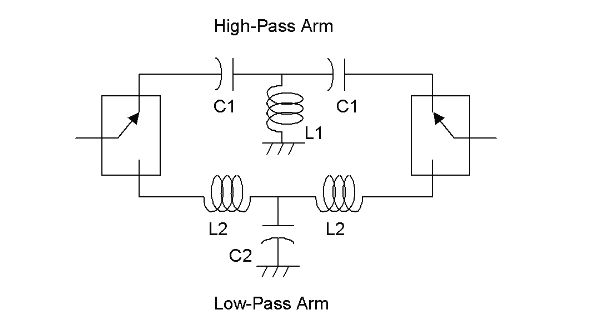High-pass/low-pass phase shifter topology with tee networks

By the way, you'll find similar schematics in a Word document you can get for free in our download area, it's called Electronic_Symbols_xx.doc, where xx is the revision number.

### Calculating exact lumped element values

In the preceding example, an optimizer was used to determine the L and C values. But you can use simple math to come up with values that will give you the exact result at center frequency, then optimize the values over your frequency band if you prefer.

You have two equivalent choices for each filter, the tee or the pi; your decision might be driven by whichever one offers a more compact layout, or perhaps one network will violate a design rule for minimum capacitor size. Below are annotated schematics to reduce confusion. Note that you could use more lumped elements than the three shown, but usually three elements will work just fine. Actually, the low-pass network can be approximated with just a high-impedance transmission line, in this case you only need one element!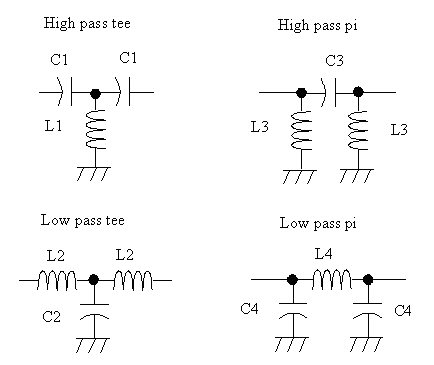Here's equations for the ideal lumped element values. For a phase bit with Φ phase shift, use Φ/2 in the equations to obtain half of the phase shift from each network. One textbook reference for these equations (in a slightly different form) is Robert Weber's Introduction to Microwave Circuits.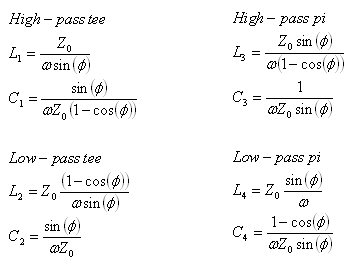Note that phase Φ is in radians when you use Excel.

Thanks to Avinash Sharma, we now have a derivation of the equations for high-pass/low-pass filters used in phase shifters. Check it out!

Below are exact lumped element values for four different bits at 6 GHz (which of course we calculated in a spreadsheet!) You can scale the L and C values as 1/frequency, or make your own spreadsheet, or wait for us to post ours one of these days. Remember that each filter provides half the desired phase shift, i.e. the LP filter for 22.5 degrees provides 11.25 degrees (negative) phase.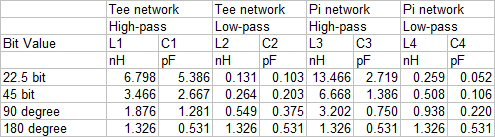Now let's compare inductor and capacitor values from the equations to the preceding example that arrived at values using an optimizer, for the 6 GHz, 90 degree bit using tee networks. They are pretty close!

 Calculated Optimized L1 1.876 nH 2.09 nH C1 1.281 pF 1.40 pF L2 0.549 nH 0.63 nH C2 0.375 pF 0.40 pF

We often get feedback from engineers on the inability to obtain inductor and capacitor values of nonstandard values. Most phase shifter designs are in monolithic microwave (MMIC) format, where you can create any value lumped element you want, subject to size constraints and the ability to deal with parasitic effects.

Now, check out our spreadsheet that performs the lumped element value calculations and plots the response over frequency!

### Lumped element phase shifter example 1

Let's look at a MMIC phase shifter that employs the lumped element technique at 35 GHz, Qorvo's TGP 2104. While we're on the subject, we write "a MMIC" instead of "an MMIC" because we pronounce the acronym "mimic", not "M-M-I-C", can you IEEE cats dig that?

Back-to-back switches are comprised of series switch FETs with the gate fingers aligned vertically. By floating the FETs to +5 volts (supplied by pad "V2") the control voltage (V1) is positive (0 or 5 volts) instead of the normal negative voltage associated with switch FETs. There is a lot of stuff you can't see in the die map, including several high-value resistors that complete the bias network; somewhere there must be an "inverter" to supply complimentary logic to two of the switch FETs; you can't see the blocking caps on the input and output RF lines, they are built into the RF paths. There are visible ESD protection diodes at each of the bias pads, connected to the ground vias of the right side RF probe point.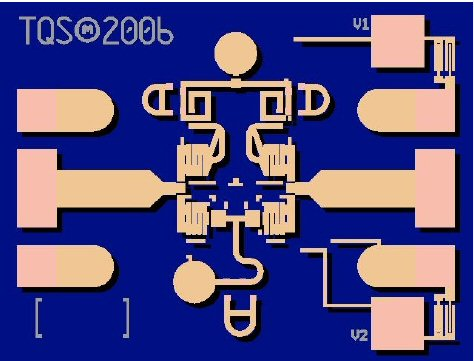The low-pass network is on the north side of the chip and uses the tee topology, the high-pass network is on the south side and also uses the tee topology. The calculated values for L1, C1, L2 and C2 are 0.227 nH, 0.091 pF, 0.227 nH and 0.091 pF respectively. Did we mention that for the ideal 180 bit calculation, the L's and C's are the same for the HP and LP networks?

In many real phase shifters you'll find that more than 50% of the phase shift is done in the low pass network and less than 50% in done in the high pass. Why? It just seems to give better bandwidth! That is exactly what is going on in the TGP2104. The series inductors in the LP network are longer than the shunt inductor in the HP network. So the LP network contributes perhaps -135 degrees phase shift while the HP network contributes +45 degrees.

This phase shifter bit has about 4 dB loss which is undesirable but understandable. This loss is caused primarily by the switch FETs. As you go up in millimeterwave frequencies, switch FET sizes must be reduced to minimize their parasitic off-capacitance, which increases their on-resistance.

So, what are those three tiny trombones on the inductors? These allow Qorvo engineers to mess around with the design in the lab to optimize the response by cutting and smooshing metal. Even though they own the GaAs foundry and the models, they often resort to this tedious trick. You'd think that they would remove the leftovers before they create a production mask (or data sheet.) Way to go, instilling modeling confidence for the rest of your foundry customers!

Author : Unknown Editor